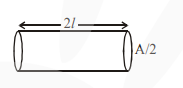# A conducting wire of length 'l',`
Question:

A conducting wire of length 'l', area of crosssection A and electric resistivity U is connected between the terminals of a battery. A potential difference V is developed between its ends, causing an electric current.

If the length of the wire of the same material is doubled and the area of cross-section is halved, the resultant current would be :

1. $\frac{1}{4} \frac{\mathrm{VA}}{\rho l}$

2. $\frac{3}{4} \frac{\mathrm{VA}}{\rho l}$

3. $\frac{1}{4} \frac{\rho l}{\mathrm{VA}}$

4. $4 \frac{\mathrm{VA}}{\rho l}$

Correct Option: 1,

Solution:

As per the questionResistance $=\frac{\rho(2 l)}{(\mathrm{A} / 2)}=\frac{4 \rho l}{\mathrm{~A}}$

$\Rightarrow$ Current $=\frac{V}{R}=\frac{V A}{4 \rho l}$